Скачать презентацию AN-NAJAH NATIONAL UNIVERSITY FACULTY OF ENGNEERING ELECTRICAL DEPARTMENT

d6a7f12d4ffd2a2ce61e52b3f00f2d83.ppt

• Количество слайдов: 17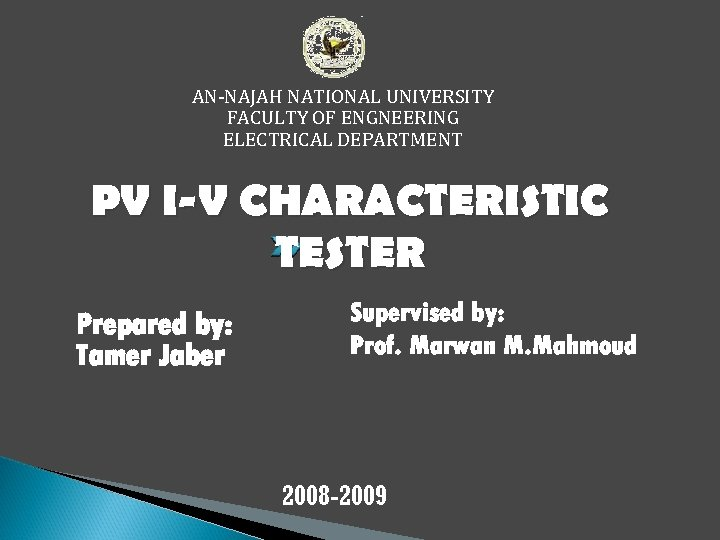AN-NAJAH NATIONAL UNIVERSITY FACULTY OF ENGNEERING ELECTRICAL DEPARTMENT PV I-V CHARACTERISTIC TESTER Prepared by: Tamer Jaber Supervised by: Prof. Marwan M. Mahmoud 2008 -2009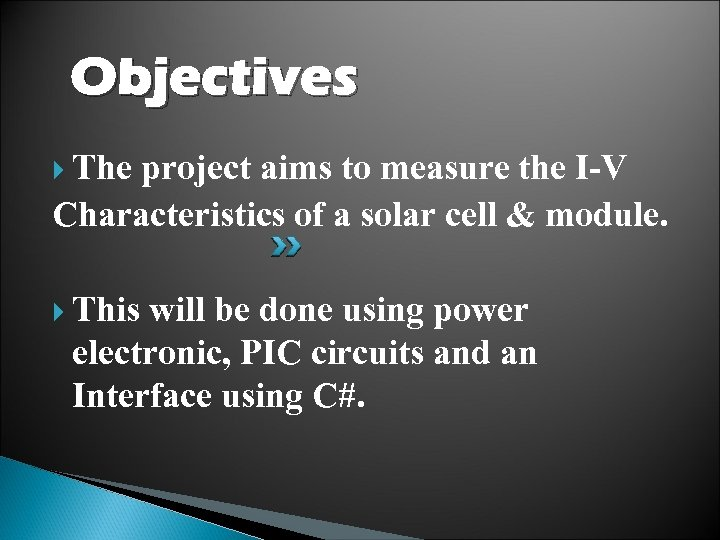Objectives The project aims to measure the I-V Characteristics of a solar cell & module. This will be done using power electronic, PIC circuits and an Interface using C#.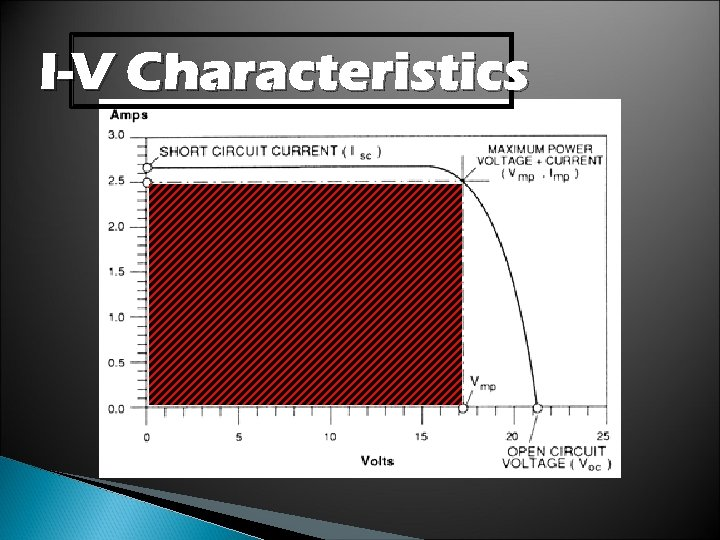I-V Characteristics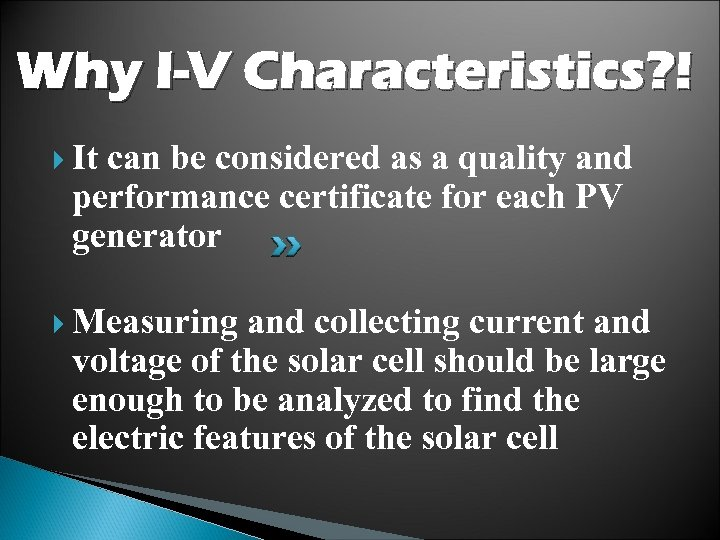Why I-V Characteristics? ! It can be considered as a quality and performance certificate for each PV generator Measuring and collecting current and voltage of the solar cell should be large enough to be analyzed to find the electric features of the solar cell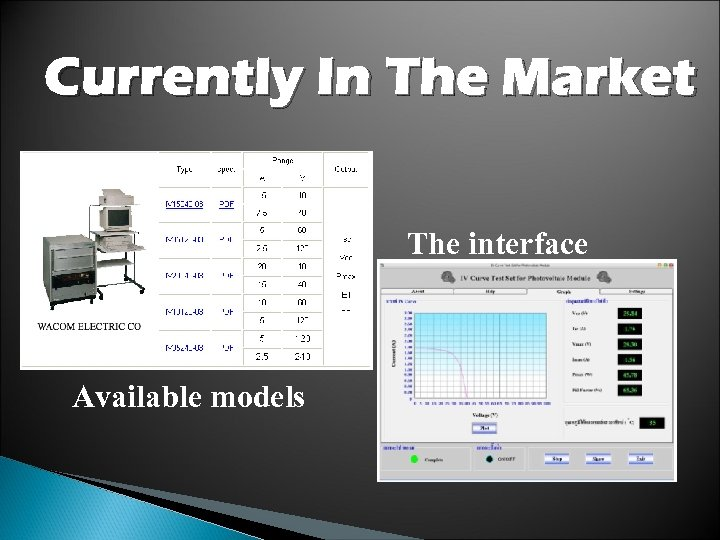Currently In The Market The interface Available models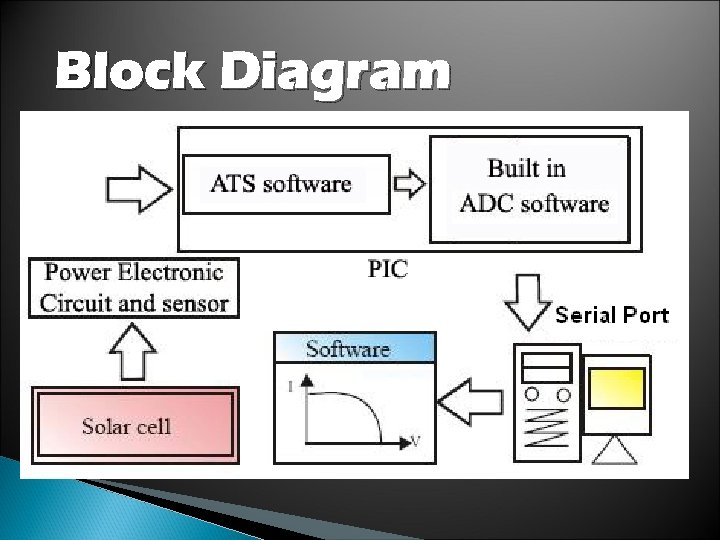Block Diagram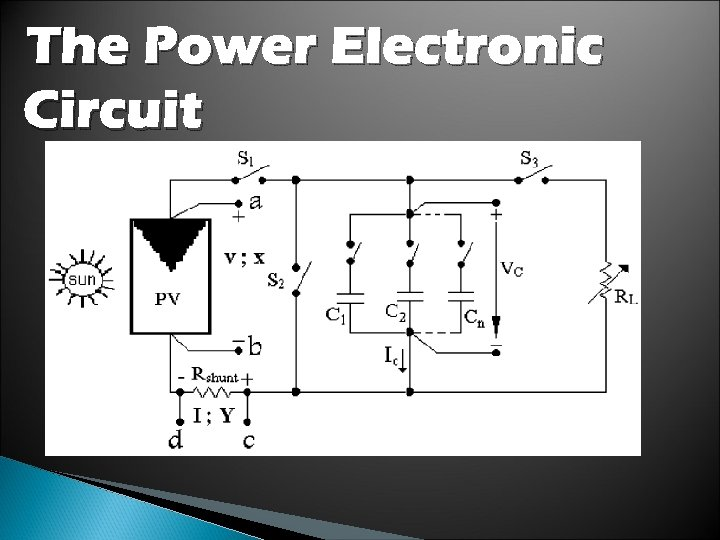The Power Electronic Circuit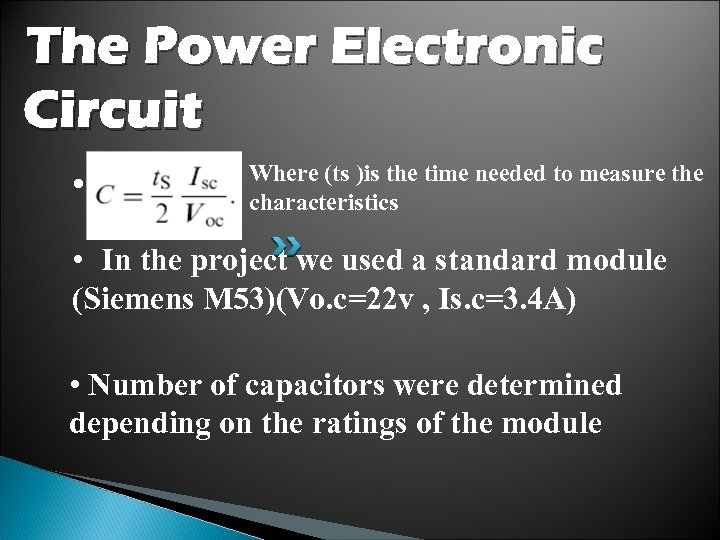The Power Electronic Circuit • Where (ts )is the time needed to measure the characteristics • In the project we used a standard module (Siemens M 53)(Vo. c=22 v , Is. c=3. 4 A) • Number of capacitors were determined depending on the ratings of the moduleThe Power Electronic Circuit • • Switches shown can be manual or automatic (transistors or relays) • RL to discharge the capacitors via S 3 • Rshunt to enable us to determine the current from voltage.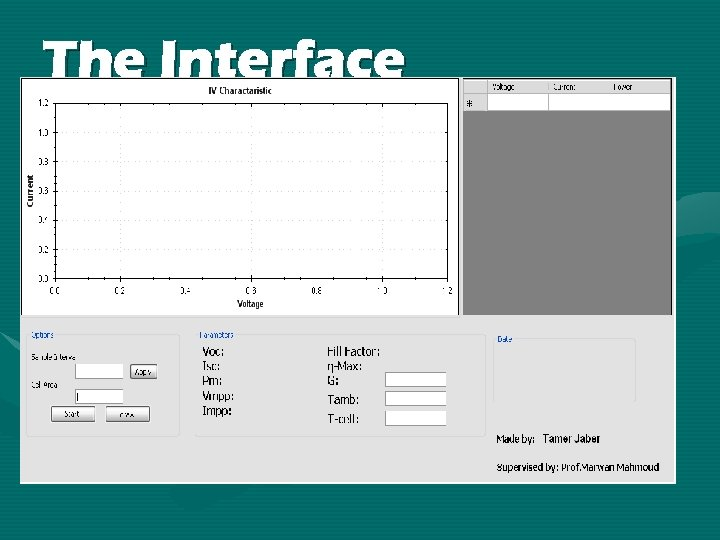The Interface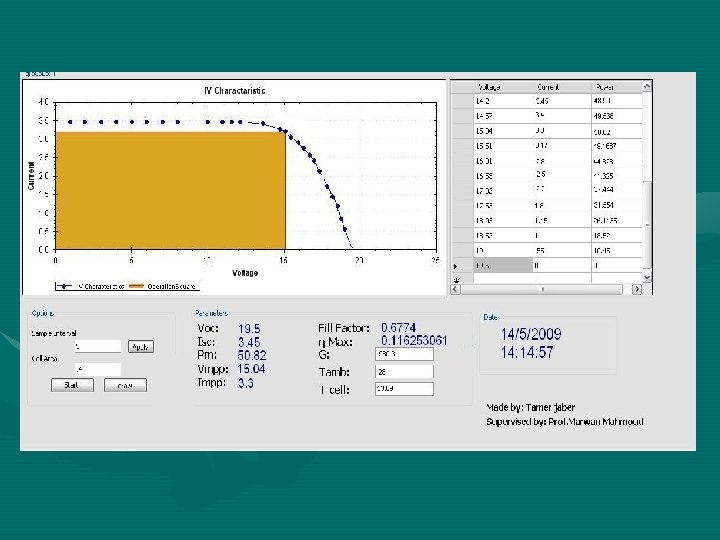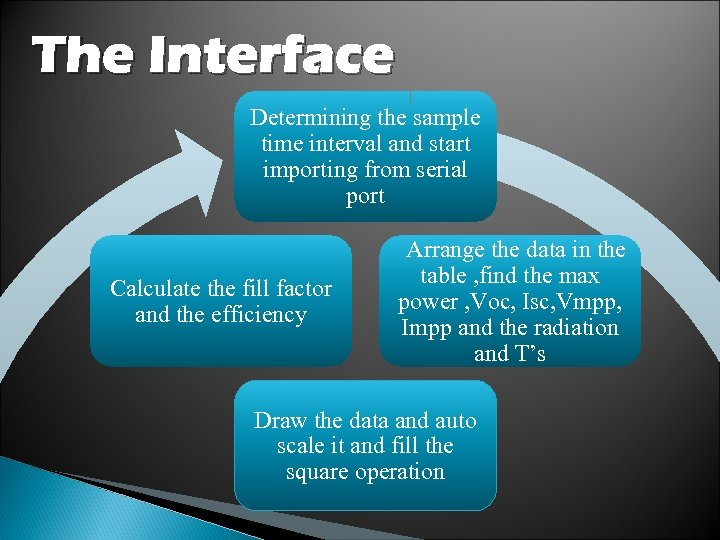The Interface Determining the sample time interval and start importing from serial port Calculate the fill factor and the efficiency Arrange the data in the table , find the max power , Voc, Isc, Vmpp, Impp and the radiation and T’s Draw the data and auto scale it and fill the square operation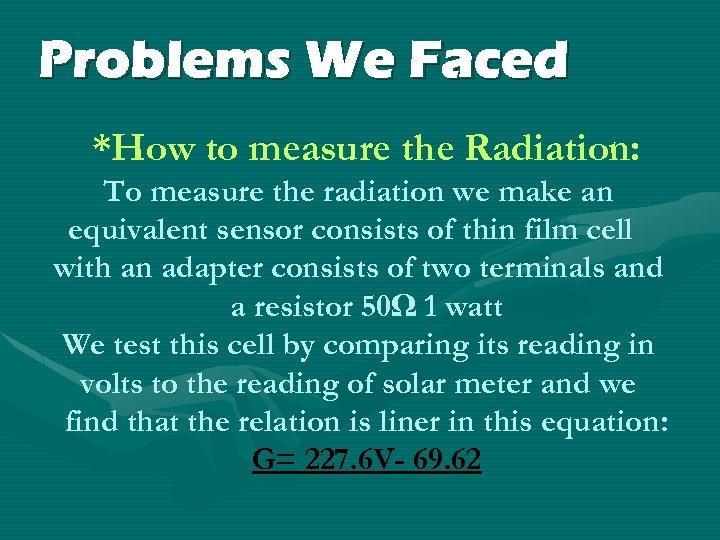Problems We Faced *How to measure the Radiation: To measure the radiation we make an equivalent sensor consists of thin film cell with an adapter consists of two terminals and a resistor 50Ω 1 watt We test this cell by comparing its reading in volts to the reading of solar meter and we find that the relation is liner in this equation: G= 227. 6 V- 69. 62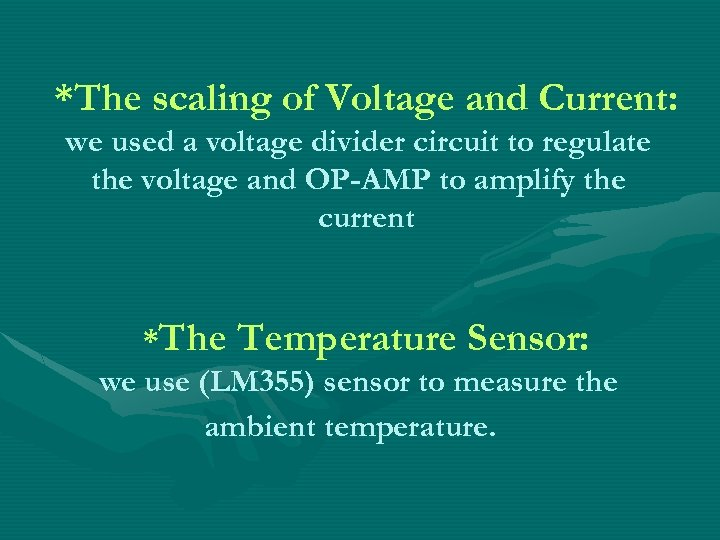*The scaling of Voltage and Current: we used a voltage divider circuit to regulate the voltage and OP-AMP to amplify the current *The Temperature Sensor: we use (LM 355) sensor to measure the ambient temperature.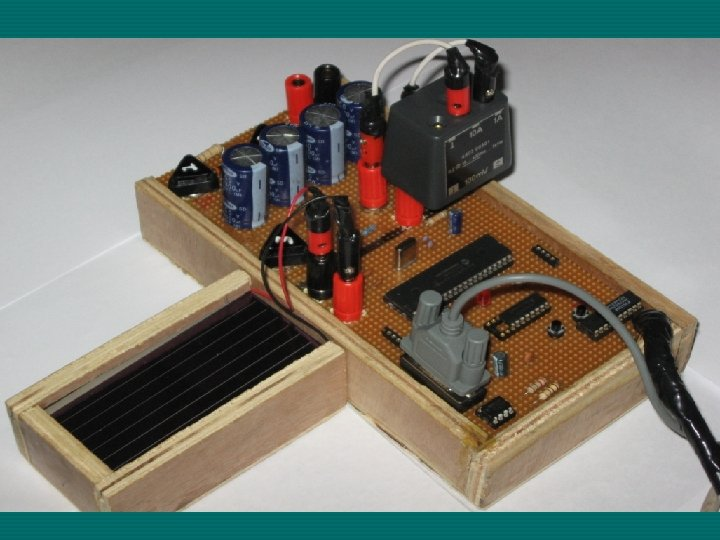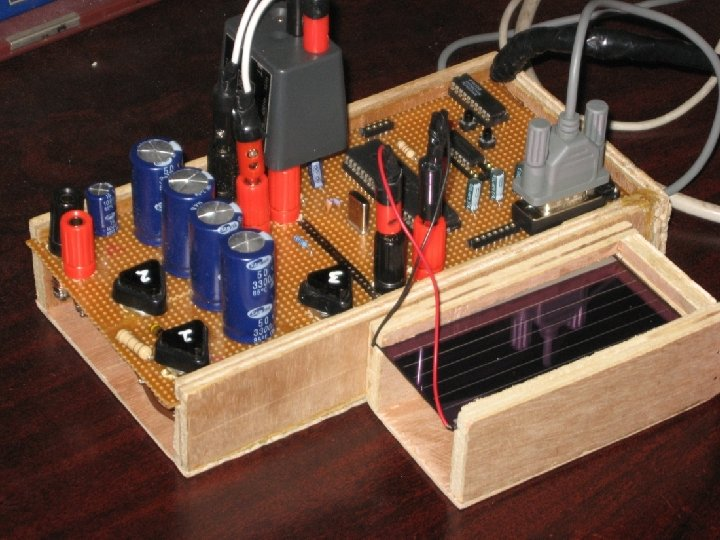Thank You